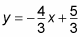##### Pre-Calculus For DummiesIn pre-calculus, you may need to find the equation of asymptotes to help you sketch the curves of a hyperbola. Because hyperbolas are formed by a curve where the difference of the distances between two points is constant, the curves behave differently than other conic sections. This figure compares the different conic sections.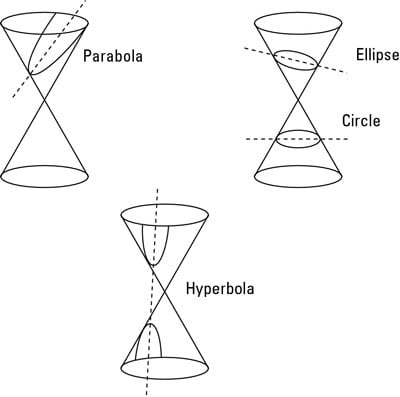Cutting the right cone with a plane to get conic sections.
Because distances can't be negative, the graph has asymptotes that the curve can't cross over.Creating a rectangle to graph a hyperbola with asymptotes.

Hyperbolas are the only conic sections with asymptotes. Even though parabolas and hyperbolas look very similar, parabolas are formed by the distance from a point and the distance to a line being the same. Therefore, parabolas don't have asymptotes.

Some pre-calculus problems ask you to find not only the graph of the hyperbola but also the equation of the lines that determine the asymptotes. When asked to find the equation of the asymptotes, your answer depends on whether the hyperbola is horizontal or vertical.

If the hyperbola is horizontal, the asymptotes are given by the line with the equation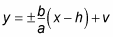If the hyperbola is vertical, the asymptotes have the equation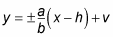The fractions b/a and a/b are the slopes of the lines. Now that you know the slope of your line and a point (which is the center of the hyperbola), you can always write the equations without having to memorize the two asymptote formulas.

You can find the slope of the asymptote in this example,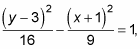by following these steps:

1. Find the slope of the asymptotes.

The hyperbola is vertical so the slope of the asymptotes is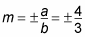2. Use the slope from Step 1 and the center of the hyperbola as the point to find the point-slope form of the equation.

Remember that the equation of a line with slope m through point (x1, y1) is y – y1 = m(x – x1). Therefore, if the slope is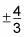and the point is (–1, 3), then the equation of the line is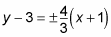3. Solve for y to find the equation in slope-intercept form.

You have to do each asymptote separately here.

• Distribute 4/3 on the right to get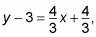and then add 3 to both sides to get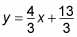• Distribute –4/3 to the right side to get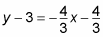Then add 3 to both sides to get